Module Segmentation
opals::ISegmentation

# Aim of module

Provides methods for point cloud segmentation based on local homogeneity using either a generic region growing approach or an adapted region growing for plane extraction.

# General description

As the single points in the point cloud do not contain information about the connectivity and structure of scanned objects, segmentation is needed to group points with similar properties. This module provides two different segmentation methods, that are both based on the seeded region growing approach. In both segmentation modes, a proper homogeneity criterion allows to detect segments for objects of interest.
The used seeded region growing approach works as follows (see Figure 1):

1. Pick random seed point for new (initial) segment
2. Check homogeneity between the seed point and its neighbouring points
3. Every neighbouring point that fulfils the criterion gets added to the list of seed points of a segment. Subsequently, each added segment point will be selected as seed point for the region growing and performs its local homogeneity check (-> go to 2).
4. If all points in the list of seed points were processed, which means no new points can be added to the segment, the segment will be closed.
5. The segmentation continues with the remaining points, that do not belong to segments yet, (-> go to 1) until all points are processed.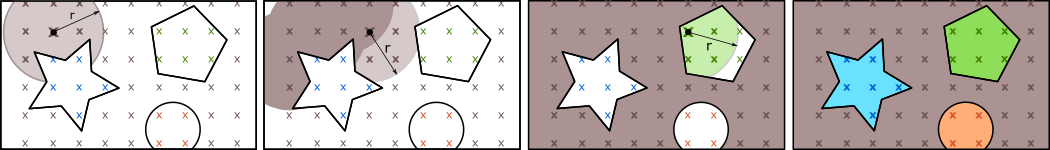Fig. 1: Seeded Region Growing

The point cloud is read from an OPALS Data Manager (ODM) input file (parameter inFile). Because of the tile based data structure of the ODM the segmentation is executed in two main stages:

• Segmentation on each tile independently
• Merge of the segments from different tiles

The final segment ids of the points are written to the ODM predefined attribute SegmentID.
Because of noise in the data some very small segments occur in the segmentation process. In most cases we are not interested in these tiny "noise" segments, thus a minimum segment size (minSegSize) can be specified (default=100 points). The segment id is only assigned to segments with at least minSegSize points. For smaller segments the SegmentID is set to null.

## Conditional Clustering - Homogeneity criterion

The conditional clustering (default segmentation mode) computes segments of points that have local homogeneity, defined by a generic filter (Parameter condClustering.criterion). The generic filter syntax allows a combination of different predefined and user-defined attributes. Local homogeneity is given, if (in)equations or other logical conditions between neighbouring points result in a true condition. More precisely, a neighbouring point n of a seed point p is added to the segment if the check of the homogeneity condition P (condClustering.criterion) returns true:

$$\LARGE P(p,n) = TRUE$$

Point attributes can be accessed for the seed point as well as for the neighbour point (n) (see Examples).
To get meaningful results in the seeded region growing, the criterion should test a local relationship between seed point and neighbouring point in a symmetric way. Only if the homogeneity criterion is symmetric, which implies that the check of the criterion P delivers the same result in both directions for a point p and its neighbour point n, the result is independent of the ODM tile size and the selection of the seed points.

$$\LARGE P(p,n) = P(n,p)$$

If a non-symmetric criterion is chosen, the results of several runs of the segmentation may differ because of the random seed point selection.

## Plane Extraction

This alternative segmentation mode aims to detect planar surfaces from point clouds with normal vector information. Unlike the generic conditional clustering, this approach does not use the criterion string, as the homogeneity criterion is given by a coplanarity condition. In the plane extraction mode a neighbouring point n of a seed point p is added to the segment if it fits well to the adjusted segment plane. To determine whether the neighbouring point fits well enough to the plane, two threshold values need to be passed:

• Distance of the point to the plane is less than planeExtraction.maxDist
• Angle between the normal vector of the point and the normal vector of the plane is within $$max(2 \cdot atan(\text{NormalSigma0}), 10 \text{deg})$$

The adjusted segment plane gets updated gradually as new points are added to the segment. The plane parameters are only updated if the standard deviation of the plane adjustment for all segment points is lower than planeExtraction.maxSigma. This prevents, that the plane moves from one planar area to another, resulting in a wrong segment.

A major difference to the default conditional clustering is, that the seed point order plays an important role for the detection of planar segments. While the seed points can be randomly selected in the conditional clustering (with a symmetric criterion), the planar surface extraction needs initial seed points that lie within a planar area to get stable initial plane parameters for a segment. To select appropriate initial seed points the parameter planeExtraction.seedCalculator can be used. By default the planeExtraction.seedCalculator sorts the points by the standard deviation of the normal vector calculation (NormalSigma0). In general, the generic calculator should return a weight for each point that might be initial seed point of a segment. Based on the returned point weight the seed points are sorted in ascending order. This means, that the region growing starts at points with a low weight, which are assumed to be in planar areas.

Note: To use the plane extraction on a point cloud, opalsNormals needs to be run first.

Alpha shapes: For all segments concave planar hulls (= $$\alpha$$-shapes) can be computed by setting a valid alphaRadius. The alphaRadius specifies the degree of generalization of the concave hull. Additional information about $$\alpha$$-shapes can be found in the documentation of the module opalsBounds. The $$\alpha$$-shapes of a segment can be can be accessed via C++ (demoSegmentation.cpp) and Python (demoSegmentation.py) API, as shown in the examples. Based on $$\alpha$$-shapes and additional segment attributes a geometric analysis of segments can be carried out.

# Parameter description

-inFileodm input file
Type: opals::Path
Remarks: mandatory
Specifies the opals datamanager (ODM) input file.
-methodsegmentation method
Type: opals::SegmentationMethod
Remarks: default=condClustering
Possible values:
condClustering .... Performs the default conditional region growing based on euclidean 2D, 2.5D or 3D neighbourhood search
planeExtraction ... This method detects planar surface segments with the use of normal vectors

Choose between conditional clustering and plane extraction mode.
Type: opals::Vector<float>
Remarks: mandatory
All points within the search area (search neighbourhood) are considered in the local homogeneity check.Depending on the parameter searchMode the size/shape of the search query object can be defined. Wheras infinte cylinder (d2) and sphere (d3) queries take a single search radius only, cylinder (d2_5) queries accept up to three distance values: radius of cylinder, dzmin (relative height of the cylinders bottom; usually negative value), dzmax (relative height of the cylinders top). If only the cylinder radius is defined, dzmin is set to -radius and dzmax to radius. In case of two values, the cylinder radius is set to value(1), dzmin to -value(2) and dzmax to value(2).
-searchModedimension of the point selection
Type: opals::SearchMode
Remarks: default=d3
Possible values:
d2 ..... Search based on 2D coordinates (x and y) only
d3 ..... Search based on full 3D coordinates (x,y and z)
d2_5 ... Search based on 3D coordinates but with different horizontal and vertical scales

The segmentation method searches neighbouring points within a infinte cylinder (d2), a finite cylinder (d2_5) or a sphere (d3).
-minSegSizeminimum point per segment
Type: uint32
Remarks: default=100
This parameter specifies the minimum number of points per segment. Only segments with at least minSegSize points are valid and thus points in smaller segments do not get a segment id.
-filterfilter strings
Type: opals::Vector<opals::String>
Remarks: optional
One or two filter strings in EBNF syntax (as described in section 'Filter syntax') can be passed to restrict the set of input points. The first filter specifies which take part in the segmentation process (if not specified, segment computation is carried out for all ODM points). The second filter defines the points that can be used as initial seed points of a segment.If not specified, any ODM point might be processed as initial segment seed point.
-condClusteringIGroup: Options for conditional clustering
These parameters are only used in the conditional clustering segmentation method
.criterionhomogeneity criterion
Type: opals::String
Remarks: default=true
Generic filter that specifies the local homogeneity criterion for the conditional region growing. The neighbour point can be accessed as "n" (see Examples). This parameter is not used in the plane extraction mode
.alphaShapeRefPlanereference plane for alpha shapes
Type: opals::Vector<double>
Remarks: optional
This optional parameter enables to specify a reference plane for the projection of the alpha shape. The projection center is the center of gravity of the segment. This parameter is only used if the parameter alphaRadius is set.If no reference plane is set, a plane is adjusted using all points of the segment
-planeExtractionIGroup: Options for plane extraction
These parameters are only used in the plane extraction mode.
.maxDistMaximum point to plane distance
Type: double
Remarks: default=0.05
This parameter defines the maximum distance of a point to the adjusted segment planeto be added to the segment.
.maxSigmaMaximum sigma0 for a segment in the plane adjustment
Type: double
Remarks: default=0.05
The adjusted plane, which represents a segment, gets updated from time to time. The plane parameters get updated if the new adjusted plane fits well enough (sigma0 < maxSigma).
.seedCalculatorCalculator strings to get seed point order
Type: opals::String
Remarks: default=NormalSigma0 < 0.02 ? NormalSigma0 : invalid
This calculator should return a numerical value (weight) for each point, which can be used for the calculation of the seed point order in the segmentation process.The seed points are sorted in ascending order of the numerical value of the calulation.For the conditional clustering the points are always selected randomly (result is independent of seed point order). For the plane extraction the seed points are ordered by the NormalSigma0 value by default.
Type: double
Remarks: optional
This optional parameter enables the computation of concave planar hulls for each segment based on the given alpha shape radius. If no alpha shape radius is specified, no alpha shape is computed. The alpha shape is projected onto the (best fitting) plane of the segment.
-sortsorting of segments
Type: opals::SegmentSort
Remarks: default=native
Possible values:
native ............ internal continous segment numbers
ascendingPSize .... sort ascending by points per segment
descendingPSize ... sort descending by point per segment

Sets the sorting method of the segments. The parameter as not influence on the segmentation itself but on the segment ids. First segment id is 0.
-debugOutFileodm output file containing segment bounding boxes
Type: opals::Path
Remarks: optional
The segment bounding boxes get written to the opals datamanager (ODM) at the given file path. Note: Existing odm-files will be overwritten.
-segmentsgets segment manger
Type: opals::SharedPtr<opals::ISegmentManager>
Remarks: output
The segment manager provids direct access to all segments, the segment points and all segment properties

# Examples

The data used in the following examples can be found in the \$OPALS_ROOT/demo/ directory. As a prerequisite the data of strip21.laz and railway.laz are imported into an OPALS data manager and the surface normal vecotrs are derived using the following commands:

opalsImport -inFile strip21.laz -tileSize 100 -filter "generic[z>265]"
opalsNormals -inFile strip21.odm -normalsAlg simplePlane -neighbours 8 -searchMode d2 -searchRad 1

The dataset (residential area) contains points on bare ground, house roofs, power lines, cars and vegetation.

opalsImport -inFile railway.laz
opalsNormals -inFile railway.odm

The second dataset contains an ALS point cloud that contains railway tracks and vegetation.

## Example 1

The first example uses an 5° angle difference criterion between adjacent normal vector.

opalsSegmentation -inFile strip21.odm -searchRadius 1 -minSegSize 75 -criterion "normalX*n.normalX+normalY*n.normalY+normalZ*n.normalZ>0.996"

Remark: The constant 0.996 in the above homogenity criterion approximates the cosine of 5°. Figure 2 shows the resulting 3D point cloud coloured by SegmentID.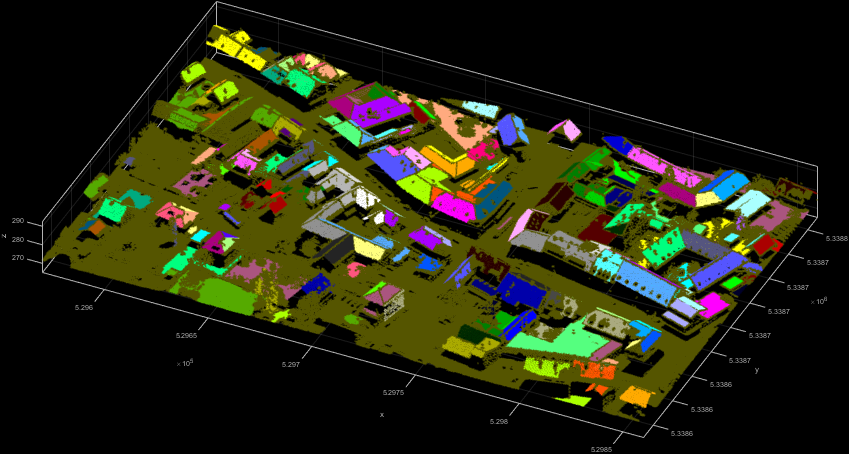Fig. 2: 3D point cloud coloured by SegmentID

## Example 2

The second example uses the difference in the z-coordinates of neighbouring points as local homogeneity criterion. Neighbouring points belong to the same segment if their difference in the z-component is below 15 cm. The resulting Figure 3 shows spatially connected roofs in the same segment.

opalsSegmentation -inFile strip21.odm -searchRadius 1 -minSegSize 100 -criterion "abs(Z-n.Z)<0.15"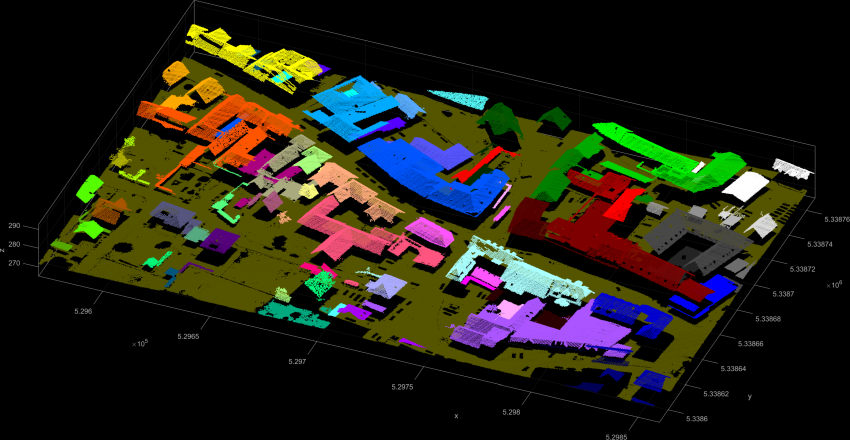Fig. 3: 3D point cloud coloured by SegmentID

## Example 3

The third example uses the railway.laz dataset to show a very basic use of the plane extraction.

opalsSegmentation -inFile railway.odm -searchRadius 0.5 -minSegSize 200 -method planeExtraction -maxDist 0.15 -maxSigma 0.1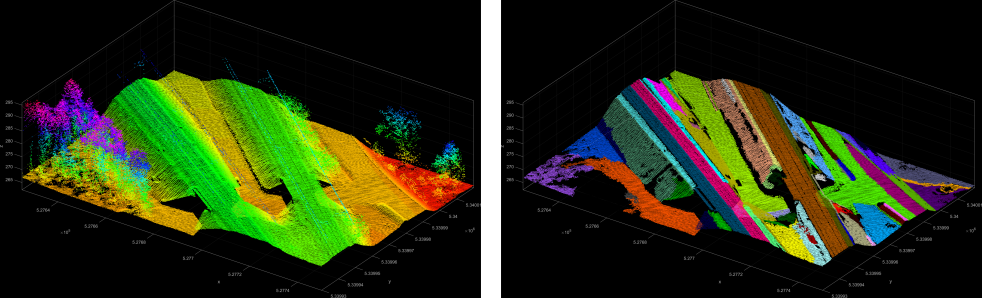Fig. 4: 3D point cloud of railway tracks (Z-coloured - left; Coloured by SegmentID - right)

## Example 4

The fourth example shows a more specific use-case for the extraction of planar segments within a given region. Segment seed points have a NormalSigma0 of less than and need to be at a height of Z > 275  m. Thus the resulting segments in Figure 5 show extracted roof faces.

opalsSegmentation -inFile strip21.odm -searchRadius 1 -minSegSize 50 -method planeExtraction -maxDist 0.2 -maxSigma 0.15 -seedCalculator "NormalSigma0<0.02 AND Z > 275 ? NormalSigma0 : invalid" -filter "Region[529568.8 5338773.5 529863 5338773.9 529862.6 5338642.4 529706 5338685.4 529569.1 5338753.8]"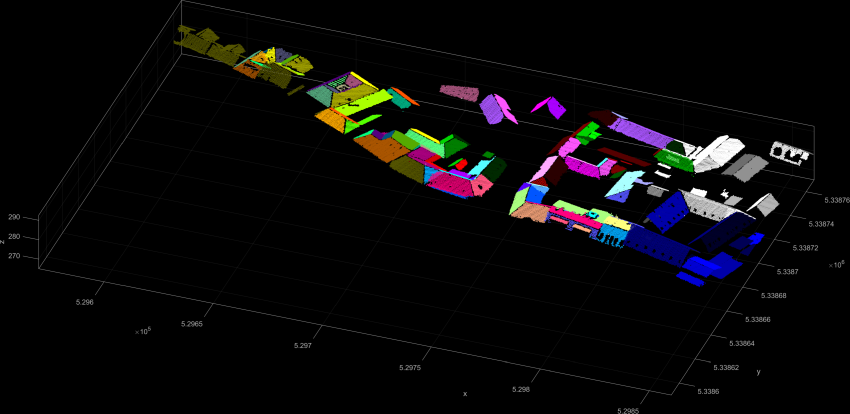Fig. 5: Roof extraction - 3D point cloud coloured by SegmentID

## Example 5: Programmatically accessing segments via the segment manager

In the examples section it is shown how the segment manager object and segment objects (including their points) can be accessed in C++ (demoSegmentation.cpp) and Python (demoSegmentation.py). These are useful features if further processing of e.g. plane parameters or segment points are desired.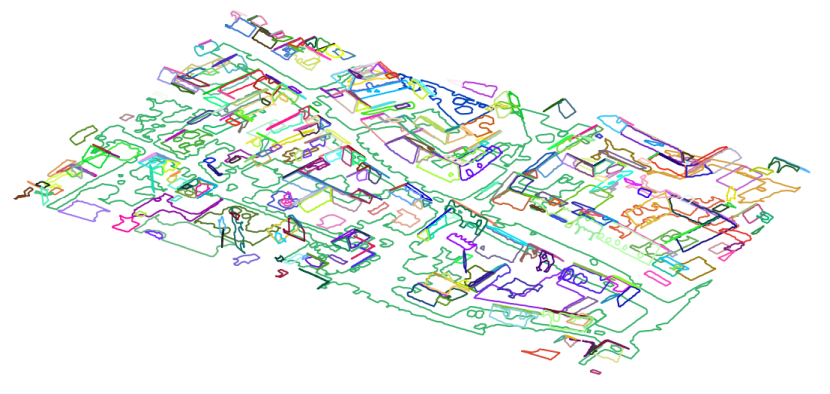Fig. 6: Visualization of alpha shapes from demoSegmentation.py

# References

Pöchtrager, M., 2016. Segmentierung Großer Punktwolken Mittels Region Growing. http://katalog.ub.tuwien.ac.at/AC13112627

Date
01.02.2019
@ d2
Search based on 2D coordinates (x and y) only.
opalsNormals is the executable file of Module Normals
Definition: ModuleExecutables.hpp:148
@ criterion
defining the segment homogeneity criterion (opalsSegmentation)
opalsSegmentation is the executable file of Module Segmentation
Definition: ModuleExecutables.hpp:193
opalsImport is the executable file of Module Import
Definition: ModuleExecutables.hpp:113
@ method
method of computation (opalsFillGaps, opalsLineTopology)
@ filter
string to be parsed in construction of DM::IFilter (various modules)
@ simplePlane
performs a simple plane fit
@ searchMode
dimension of nearest neighbor search (opalsNormals)
@ seedCalculator
Calculator for seed point order (opalsSegmentation)
@ planeExtraction
Parameter group 'planeExtraction' containing options for planar surface extraction (opalsSegmentation...
@ maxDist
maximum point distance (e.g. opalsSimplify, opalsSegmentation)